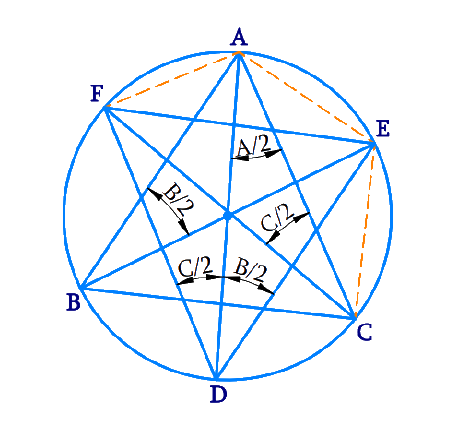# Ex.10.6 Q8 Circles Solution - NCERT Maths Class 9

Go back to  'Ex.10.6'

## Question

Bisectors of angles \begin{align} {A, B}\end{align} and \begin{align} {C}\end{align} of a triangle \begin{align} {ABC}\end{align} intersect its circumcircle at \begin{align} {D, E}\end{align} and \begin{align}{F}\end{align} respectively. Prove that the angles of the triangle \begin{align} {DEF}\end{align} are \begin {align} 90^{0}-\frac{1}{2} {A}, \,90^{\circ}-\frac{1}{2} {B},\, 90^{\circ}-\frac{1}{2} {C}\end {align}

Video Solution
Circles
Ex 10.6 | Question 8

## Text Solution

What is known?

Bisectors of angles \begin {align} {A, B} \end {align} and \begin {align} {C} \end {align} of a triangle \begin {align} {ABC} \end {align} intersect its circumcircle at \begin {align} {D, E} \end {align} and \begin {align} {F } \end {align} respectively.

What is unknown?

Proof that the angles of the triangle \begin {align} \text{DEF} \end {align} are

$90^{0}-\frac{1}{2} {A}, \;\; 90^{\circ}-\frac{1}{2} {B}, \;\; 90^{\circ}-\frac{1}{2} {C}$

Reasoning:

Angles in the same segment are equal.

Steps:It is given that \begin {align} {BE}\end {align} is the bisector of \begin {align} \angle {B.}\end {align}

\begin{align} \angle {ABE}=\frac{\angle {B}}{2} \end{align}

However,

\begin{align} \angle {ADE}&=\angle {ABE} \\\text { (Angles in} &\text{ the same} \text{ segment }\\& \text{ for chord AE })\\ \angle {ADE}&=\frac{\angle {B}}{2}\end{align}

Similarly,

\begin{align}\angle {ADF}&=\angle {ACF}\\&=\frac{\angle {C}}{2}\\\text { (Angles in} & \text{ the same} \text{ segment } \\ &\text{ for chord AF })\end {align}

\begin{align} \angle {D} &=\angle {ADE}+\angle {ADF} \\ &=\frac{\angle {B}}{2}+\frac{\angle {C}}{2} \\ &=\frac{1}{2}(\angle {B}+\angle {C}) \\ &=\frac{1}{2}\left(180^{\circ}-\angle {A}\right) \end{align}

Similarly it can be proved for

\begin{align} \angle {E} &=\frac{1}{2}\left(180^{\circ}-\angle {B}\right) \\ \angle {F} &=\frac{1}{2}\left(180^{\circ}-\angle {C}\right) \end{align}

Video Solution
Circles
Ex 10.6 | Question 8

Learn from the best math teachers and top your exams

• Live one on one classroom and doubt clearing
• Practice worksheets in and after class for conceptual clarity
• Personalized curriculum to keep up with school